# Worksheet: Polynomials Notes | Study Mathematics (Maths) Class 10 - Class 10

## Class 10: Worksheet: Polynomials Notes | Study Mathematics (Maths) Class 10 - Class 10

The document Worksheet: Polynomials Notes | Study Mathematics (Maths) Class 10 - Class 10 is a part of the Class 10 Course Mathematics (Maths) Class 10.
All you need of Class 10 at this link: Class 10

1. Find the quadratic polynomial whose zeroes are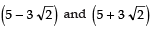respectively.
2. Find the zeroes of the polynomial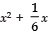– 2.
3. Divide: x3 − 1 by x + 1.
4. Find the quadratic polynomial whose zeroes are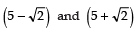respectively.
5. Divide: x4 − 1 by x + 1.
6. Divide: 4x3 − x + 1 by 2x − 1.
7. Divide: x4 + x2 + 1 by x2 + x + 1.
8. Find the quotient and remainder when x4 + 1 is divided by x − 1.
9. Find the quotient and remainder when f (x) = 4x3 − 12x2 + 14x − 13 is divided by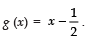10. Find the quotient and remainder when 3x4 + 5x3 –7x2 + 2x + 2 is divided by x2 + 3x + 1.
11. Find the remainder when x4 – 3x2 + 4x + 5 is divided by x2 – x + 1.
12. On dividing x3 − 3x2 + x + 2 by a polynomial g (x), the quotient and remainder were x − 2 and − 2x + 4 respectively. Find g (x).
13. Find the zeroes of 2x4 − 3x3 − 3x2 + 6x − 2, if you know that two of its zeroes are √2 and -√2.
14. If α and β are the zeroes of the quadratic polynomial f (x) = x2 − 3x + 2, then find the value of α2 + β2.
15. It is given that 1 is one of the zeroes of the polynomial 7x − x3 − 6. Find its other zero.
Hint: (− x3 + 7x − 6) ÷ (x − 1)= − (x2 + x − 6) = − [(x + 3) (x - 2)]
∴ The other zeroes are −3 and 2.
16. Divide 2x4 − 9x3 + 5x2 + 3x − 8 by x2 − 4x + 1 and verify the division by algorithm.
Hint: (2x4 − 9x3 + 5x2 + 3x − 8) ÷ (x2 − 4x + 1)
⇒ quotient = 2x2 − x − 1 and remainder = −7
Verification:
(Quotient × Divisor) + Remainder = Dividend or (2x2 − x − 1) × (x2 − 4x + 1) + (− 7) = 2x4 − 9x3 + 5x2 + 3x − 8
17. Find k so that x2 + 2x + k is a factor of 2x4+ x3 – 14x2 + 5x + 6. Also, find all the zeroes of the polynomial.
Hint: For (x2 + 2x + k) to be a factor the remainder in (2x4 + x3 – 14x2 + 5x + 6) ÷ (x2 + 2x + k) must be zero.
18. If α, β are the zeroes of the quadraticpolynomial p(x) = x2 – (k–6)x + (2k + 1), find the value of k when α + β = αβ.
Hint: Sum of roots α + β = –[–(k – 6)]= k – 6
Product of roots αβ = 2k + 1
∵ α + β = αβ
∴ k – 6 = 2k + 1
⇒ (2k– k) = – 6 – 1
∴ k = –7
19. Find the zeroes of the polynomial p(x) =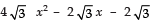and verify the relationship between the zeroes and the coefficients.
Hint: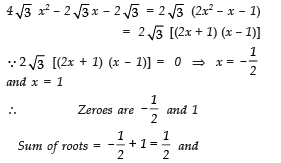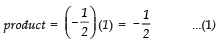In the given polynomial,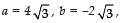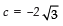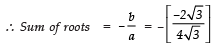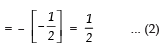Product of roots
From (1), (2) and (3), the relation between the zeroes and coefficient is verified.

1. x2 – 10x + 7
2.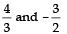3. x2 – x + 1; Remainder = –2
4. x2 – 10x + 21
5. x3 + x2 + x + 1; Remainder = – 2
6. 2x2 + x; Remainder = 1
7. x2 – x + 1; Remainder = 0
8. x3 + x2 + x + 1; Remainder = 2
9. 4x2 – 10x + 19, Remainder = -7/2
10. 3x2 – 4x + 2; Remainder = 0
11. Quotient = x2 + x –3; Remainder = 8
12. g(x) = x2 – x + 1
13.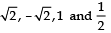14. 5
15. –3 and 2
16. 2x2 – x – 1; Remainder = – 7
17. k = – 3; zeroes;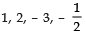18. k = –7,
19.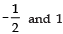The document Worksheet: Polynomials Notes | Study Mathematics (Maths) Class 10 - Class 10 is a part of the Class 10 Course Mathematics (Maths) Class 10.
All you need of Class 10 at this link: Class 10Use Code STAYHOME200 and get INR 200 additional OFF

## Mathematics (Maths) Class 10

53 videos|369 docs|138 tests

Track your progress, build streaks, highlight & save important lessons and more!

,

,

,

,

,

,

,

,

,

,

,

,

,

,

,

,

,

,

,

,

,

;